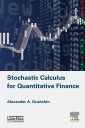• A
• A
• A
• ABC
• ABC
• ABC
• –ź
• –ź
• –ź
• –ź
• –ź
Regular version of the site

## Stochastic Calculus for Quantitative Finance

L.; Kidlington: ISTE, Elsevier, 2015.

In 1994 and 1998 F. Delbaen and W. Schachermayer published two breakthrough papers in which they proved continuous-time versions of the Fundamental Theorem of Asset Pricing. This is one of the most remarkable achievements in modern Mathematical Finance which led to intensive investigations in many applications of the arbitrage theory on a mathematically rigorous basis of stochastic calculus.

This book provides the reader with a detailed understanding of all necessary attributes in stochastic calculus that are required for applications of the theory of stochastic integration in Mathematical Finance, in particular, in the arbitrage theory. The exposition follows the traditions of the Strasbourg school.